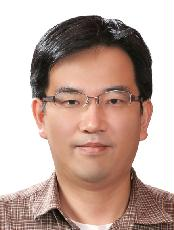###정보이름최영우 학력박사 전공수학전공(과) 연구실 : 팔달관718호 연구실번호 : 2570 이메일 : youngwoo@ajou.ac.kr 연구관심분야 : 조화해석학 홈페이지1 : 홈페이지2 : 졸업연도/학교/학위 1995.12 University of Wisconsin-Madison 박사 졸업연도/학교/학위 1991.02 서울대학교 학사 연구활동(주요논문) [논문] 최영우, 정재원, Totally interpolating multiwavelet systems with approximation order , Linear and Multilinear Algebra , Vol.60 , No.6 , pp.697 -724 (Jun, 2012) [논문] 최영우, The L^p-L^q mapping properties of convolution operators with the affine arclength measure on space curves , Journal of the Australian Mathematical Society , Vol.75 , No.1 , pp.247 -261 (Oct, 2003) [논문] 최영우, Marcinkiewicz integrals with rough homogeneous kernels of degree zero in product domains , Journal of Mathematical Analysis and Applications , Vol.261 , No.1 , pp.53 -60 (Aug, 2001) [논문] 최영우, Damping oscillatory integrals with polynomial phase and convolution operators with the affine arclength measure on polynomial curves in R^n , Journal of the London Mathematical Society , Vol.61 , No.1 , pp.275 -285 (Feb, 2000) [논문] 최영우, Convolution operators with the affine arclength measure on plane curves , 대한수학회지 (J. Korean Math. Soc.) , Vol.36 , No.1 , pp.193 -207 (Jan, 1999) 국제학술논문지 [논문] 최영우, 정재원, Interpolating refinable function vectors with reflection properties , JOURNAL OF COMPUTATIONAL AND APPLIED MATHEMATICS , Vol.294 , pp.297 -308 (Mar, 2016) [논문] 최영우, Uniform Lorentz norm estimates for convolution operators , JOURNAL OF INEQUALITIES AND APPLICATIONS , Vol.2014 , No.256 , pp.1 -9 (Jul, 2014) [논문] 최영우, Uniform estimates for damped Radon transform on the plane , ABSTRACT AND APPLIED ANALYSIS , Vol.2013 , pp.1 -5 (Oct, 2013) [논문] 최영우, 정재원, Totally interpolating multiwavelet systems with approximation order , Linear and Multilinear Algebra , Vol.60 , No.6 , pp.697 -724 (Jun, 2012) [논문] 최영우, 정재원, APPROXIMATION AND BALANCING ORDERS FOR TOTALLY INTERPOLATING BIORTHOGONAL MULTIWAVELET SYSTEMS , BULLETIN OF THE KOREAN MATHEMATICAL SOCIETY , Vol.48 , No.6 , pp.1157 -1167 (Nov, 2011) [논문] 최영우, Convolution estimates related to space curves , JOURNAL OF INEQUALITIES AND APPLICATIONS , Vol.2011 , No.91 , pp.1 -6 (Oct, 2011) [논문] 최영우, The L^p-L^q mapping properties of convolution operators with the affine arclength measure on space curves , Journal of the Australian Mathematical Society , Vol.75 , No.1 , pp.247 -261 (Oct, 2003) [논문] 최영우, Marcinkiewicz integrals with rough homogeneous kernels of degree zero in product domains , Journal of Mathematical Analysis and Applications , Vol.261 , No.1 , pp.53 -60 (Aug, 2001) [논문] 최영우, Damping oscillatory integrals with polynomial phase and convolution operators with the affine arclength measure on polynomial curves in R^n , Journal of the London Mathematical Society , Vol.61 , No.1 , pp.275 -285 (Feb, 2000) 국내학술논문지 [논문] 고호경, 박선정, 최영우, 수학교육에서 빅데이터 활용 방안에 대한 소고 , 수학교육 논문집 , No.4 , pp.573 -588 (Nov, 2014) [논문] 최영우, 정재원, Infinitude of minimally supported totally interpolating biorthogonal multiwavelet systems with low approximation orders , 한국수학논문집(Korean Journal of Mathematics) , Vol.21 , No.3 , pp.247 -263 (Sep, 2013) [논문] 최영우, 임경수, Mapping properties of the Marcinkiewicz integrals on homogeneous groups , Journal of the Korean Mathematical Society , Vol.39 , No.1 , pp.1 -15 (Jan, 2002) [논문] 최영우, Convolution operators with the affine arclength measure on plane curves , 대한수학회지 (J. Korean Math. Soc.) , Vol.36 , No.1 , pp.193 -207 (Jan, 1999) 국제학술발표 [학술회의] 이기정, 박지현, 최영우, A modeling of random flux for stochastic elliptic equations , 2019 Spring Probability Workshop, Taiwan (May, 2019) [학술회의] 최영우, Endpoint Lorentz norm estimates of convolution operators with the affine arclength measure on plane curves , ICM 2014 Satellite Conference in Harmonic Analysis (Aug, 2014) [학술회의] 최영우, Averaging along curves: some endpoint estimates , 2nd East Asian Conference in Harmonic Analysis and Applications (Jul, 2014) 국내학술발표 [학술회의] 최영우, Estimates for convolution operators related to plane and space curves , Harmonic analysis and PDE (Apr, 2012) [학술회의] 최영우, Logarithmic convexity and convolution estimates related to curves , 대한수학회 가을 연구발표회 , Vol.2000 , No.1 , pp.1 -32 (Oct, 2000) [학술회의] 최영우, On Marcinkiewicz integral , 대우 워크샵 , Vol.2000 , No.1 , pp.1 -51 (Jul, 2000) [학술회의] 최영우, Convolutions with measures supported on curves , 대우워크샵 (Jul, 1998) [학술회의] 최영우, L^p-L^q estimates on convolution operators , 건국대-아주대 국제수학 심포지엄 , pp.1 -2 (May, 1998)